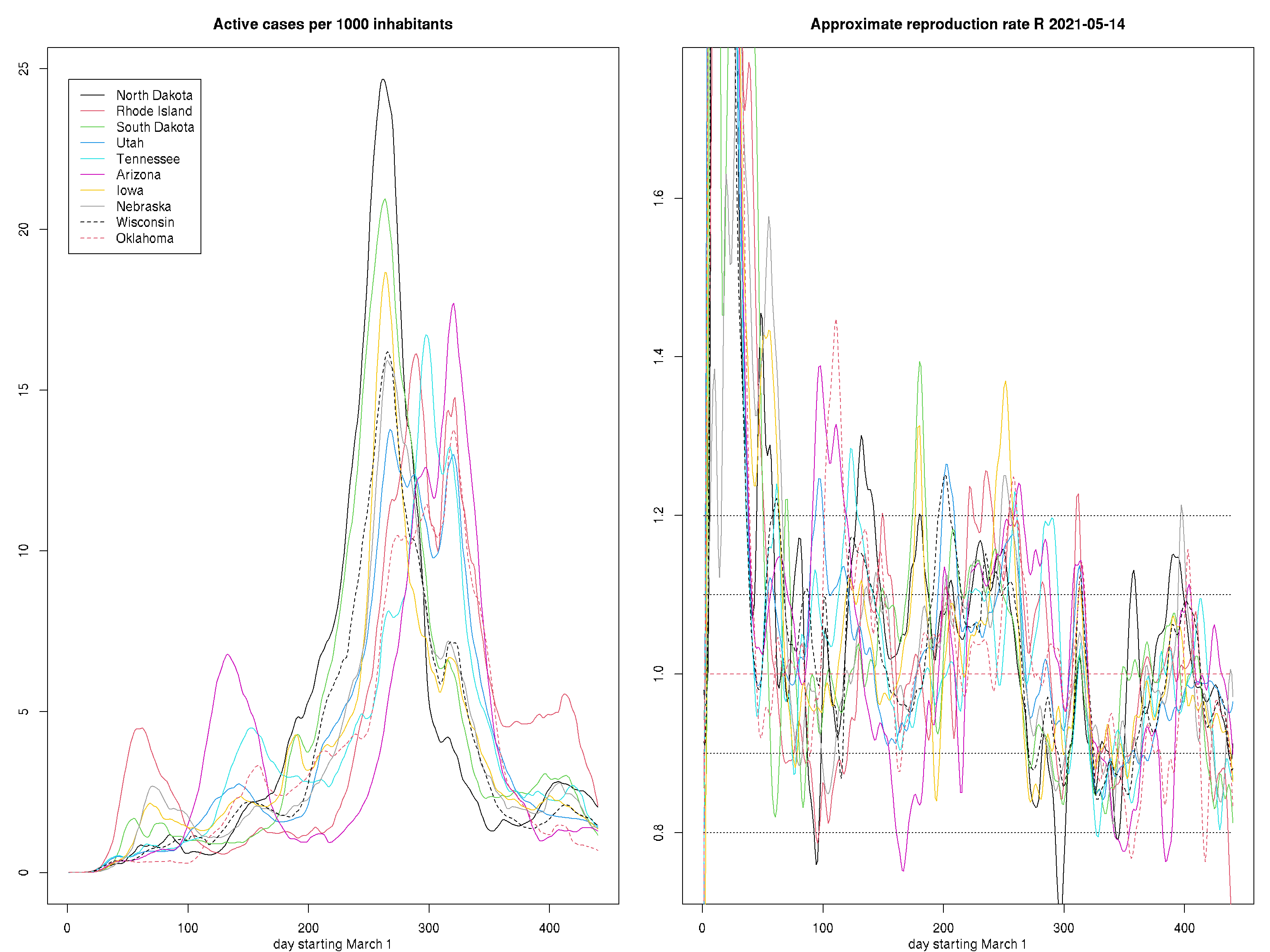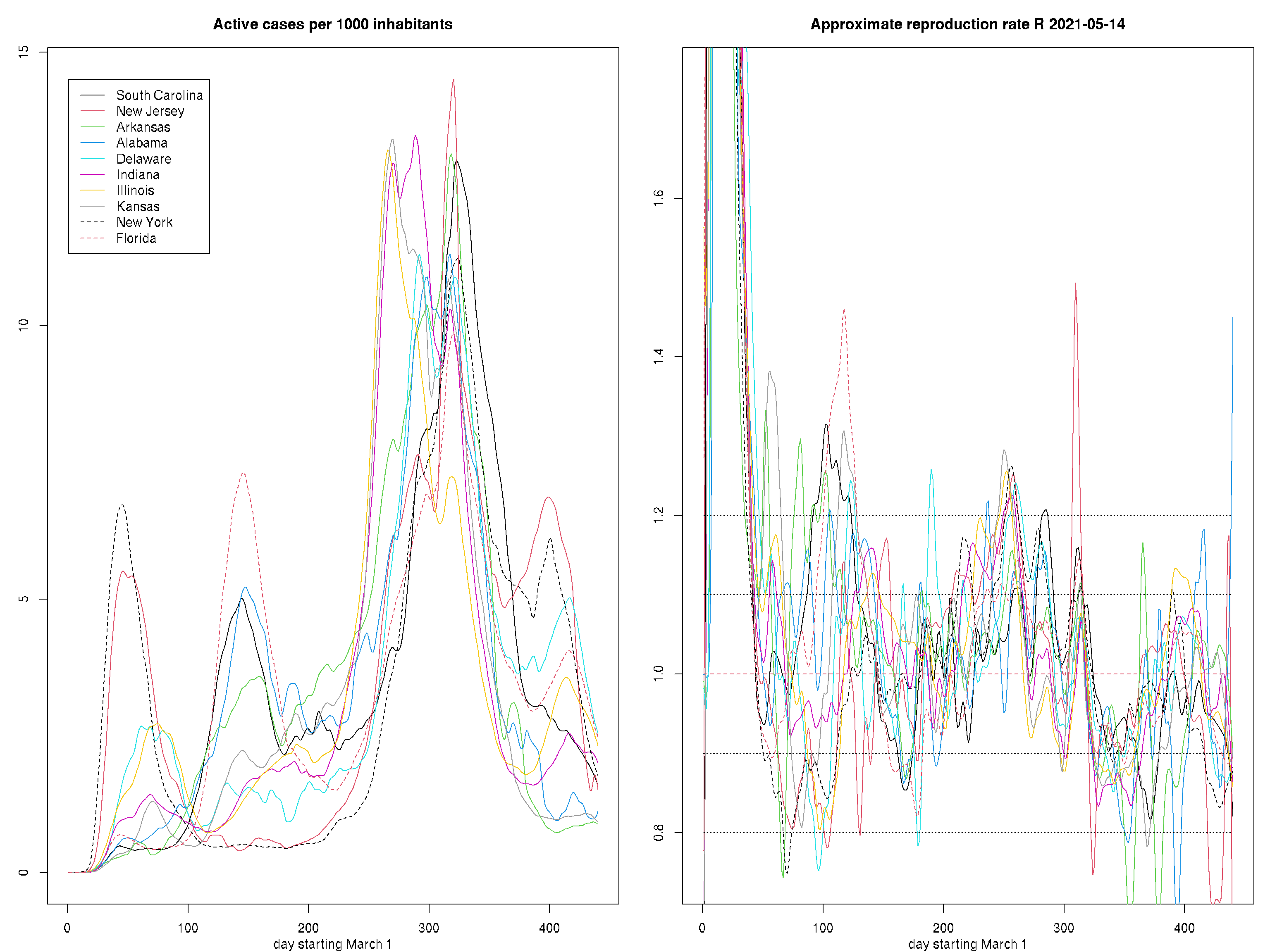## General remarks

This analysis is based on the numbers of confirmed infections and deaths provided by the New York Times at . Testing data is obtained from the covidtracking project at Johns Hopkins university, data at , for more information see .

``````data <- read.csv("https://raw.githubusercontent.com/nytimes/covid-19-data/master/us-states.csv")
states <- unique(data\$state)[c(1:50,54)]
statesabr <- unique(data2\$state)[c(53,17,6,5,22,
54,48,33,49,41,
11,38,44,12,34,
31,35,7,23,37,
47,14,18,20,26,
40,42,45,9,19,
27,52,51,8,15,
21,39,25,46,3,
10,29,36,32,56,
1,24,2,16,30,55)]
data <- data[data\$state%in%states,-3]
data2 <- data2[data2\$state%in%statesabr,-3]
nentries <- dim(data)
udates <- as.Date(unique(data[,1]))
udates <- udates[udates > "2020-02-29"]
ndates <- length(udates)
dates <- 1:ndates
nc <- length(states)
pop <- c(7614,12672,39512,7278,6950,
5822,28996,1934,3206,4218,
21478,19453,1059,10617,1360,
10488,8882,5759,6046,3080,
6833,1416,6732,4468,5640,
3957,12802,5149,706,2913,
6137,624,8536,3565,3155,
4649,11689,9987,885,3018,
974,2976,2097,762,579,
732,1344,4903,1787,1069,1792)
cases <- deaths <- matrix(0,ndates,nc)
for(i in 1:nentries){
j <- (1:ndates)[udates==as.Date(data\$date[i])]
k <- (1:nc)[states==data\$state[i]]
cases[j,k] <- data\$cases[i]
deaths[j,k] <- data\$deaths[i]
}
nentries2 <- dim(data2)
conv2date <- function(x){
year <- x %/% 10000
month <- (x %% 10000)%/%100
day <- x %% 100
as.Date(paste0(year,"-",month,"-",day))
}
udates2 <- conv2date(unique(data2[,1]))
udates2 <- udates2[udates2 > "2020-02-29"]
ndates2 <- length(udates2)
udates2 <- udates2[ndates2:1]
dates2 <- 1:ndates2
positive <- negative <- testincr <- matrix(0,ndates2,nc)
for(i in 1:nentries2){
j <- (1:ndates2)[udates2==conv2date(data2\$date[i])]
k <- (1:nc)[statesabr==data2\$state[i]]
positive[j,k] <- if(is.null(data2\$positiveIncrease[i])) 0 else data2\$positiveIncrease[i]
negative[j,k] <- if(is.null(data2\$negativeIncrease[i])) 0 else data2\$negativeIncrease[i]
testincr[j,k] <- if(is.null(data2\$totalTestResultsIncrease[i])) 0 else data2\$totalTestResultsIncrease[i]
}
positive[is.na(positive)] <- 0
negative[is.na(negative)] <- 0
tests <- pmax(0,positive) + pmax(0,negative)
tests[testincr>tests] <- testincr[testincr>tests]
dim(tests) <- dim(positive)
# take moving averages over weeks
tests <- rbind(matrix(0,7,51),apply(apply(tests,2,cumsum),2,diff,7)/7)
positive <- rbind(matrix(0,7,51),apply(apply(positive,2,cumsum),2,diff,7)/7)

posprop <-  positive/tests
posprop[is.na(posprop)] <- 0
posprop[posprop>=1] <- 0 # no negatives recorded
posprop[posprop<0] <- 0
tests[tests<0] <- 0
testincr[testincr<0] <- 0
testspermill <- sweep(testincr,2,pop,"/")*1000

deaths[120:121,5] <- 8054 # Massechusets reported -41 deaths on day 122
rate <- sweep(cases,2,pop,"/")
rate[rate < 1e-4] <- 1e-4
diffrate <- apply(rate,2,diff)
ind <- order(rate[ndates,],decreasing = TRUE)``````

For modeling we 2use local polynomial regression as implemented in function locpoly in R package KernSmooth. We employ a Gaussian kernel with bandwidth (kernel standard deviation) 1.5 days for estimating rates and 2.5 for estimating derivatives. Polynomial orders are 1 and 2, respectiviley, to minimize boundary bias. dates are in days after March 1.

Please note that the estimated curves for small number of reported cases carry a high variability. This is especially due for early dates and states with low population (ISL, EST). Problems in the data like underreporting and reporting bias around weekends are not modeled.

## Active cases and estimated effective reproduction number R

Active cases are here defined as cases that were reported within the last 14 days. This is more conservative than the rule used, e.g., by the German RKI (corresponding to approximately the last 11 days) and was chosen to minimize effects due to a weekly cycly of reporting biases observed in the German reporting data.

``````bw <- 1.5
dbw <- 2.5
inc <- 14 # this is the length of the time period used in the definition of active cases
rlcurves <- dlcurves <- list(NULL)
rlylim <- dlylim <- NULL
for (i in 1:nc) {
rlcurves[[i]]<- locpoly(dates,log(pmax(1e-4,diff(c(rep(0,inc),rate[,i]),inc))),bandwidth=bw,degree=1)
rlylim <- range(rlylim,rlcurves[[i]]\$y)
dlcurves[[i]] <- locpoly(dates,log(pmax(1e-4,diff(c(rep(0,inc),rate[,i]),inc))),bandwidth=dbw,drv=1,degree=2)
dlylim <- range(dlylim,dlcurves[[i]]\$y)
}
rlylim1 <- dlylim1 <- NULL
for(i in ind[1:10]){
rlylim1 <- range(rlylim1,rlcurves[[i]]\$y)
dlylim1 <- range(dlylim1,dlcurves[[i]]\$y)
}
rlylim2 <- dlylim2 <- NULL
for(i in ind[11:20]){
rlylim2 <- range(rlylim2,rlcurves[[i]]\$y)
dlylim2 <- range(dlylim2,dlcurves[[i]]\$y)
}
rlylim3 <- dlylim3 <- NULL
for(i in ind[21:30]){
rlylim3 <- range(rlylim3,rlcurves[[i]]\$y)
dlylim3 <- range(dlylim3,dlcurves[[i]]\$y)
}
rlylim4 <- dlylim4 <- NULL
for(i in ind[31:40]){
rlylim4 <- range(rlylim4,rlcurves[[i]]\$y)
dlylim4 <- range(dlylim4,dlcurves[[i]]\$y)
}
rlylim5 <- dlylim5 <- NULL
for(i in ind[41:51]){
rlylim5 <- range(rlylim5,rlcurves[[i]]\$y)
dlylim5 <- range(dlylim5,dlcurves[[i]]\$y)
}``````

The following figure shows quantities derived as functions of the logarithmic rate of active cases and its derivative. The latter value is closely related to R, i.e., \(R \approx exp(C*value)\) with \(C\approx 4\). Please note that what is shown is not the R reported by,regulatory b7odies like e.g., the RKI and that the value provided here is calculated from a different model. Also note that the amount and criteria of testing used differ substantially between states which makes comparisons difficult. For interpretation: keeping the effective reproduction number below 1 means that the number of active cases is decreasing.

``````par(mfrow=c(1,2),mar=c(3,3,3,1),mgp=c(2,1,0))
plot(dates,rate[,1],ylim = exp(rlylim1),type="n",xlab="day starting March 1",ylab="")
for(i in 1:10)
lines(rlcurves[[ind[i]]]\$x,exp(rlcurves[[ind[i]]]\$y),col=i,lty=1+(i-1)%/%8)
legend(1,exp(rlylim1),states[ind[1:10]],col=1:10,lty=1+(0:9)%/%8,lwd=rep(1,10))
title("Active cases per 1000 inhabitants")

plot(dates,c(0,diffrate[,1]),ylim = c(0.75,1.75),type="n",xlab="day starting March 1",ylab="")
for(i in 1:10) lines(dlcurves[[ind[i]]]\$x,exp(4*dlcurves[[ind[i]]]\$y),col=i,lty=1+(i-1)%/%8)
title(paste("Approximate reproduction rate R",as.character(udates[ndates])))
lines(range(dates),c(1,1),lty=2,col=2)
for(lvl in seq(.5,1.2,.1)[-6]) lines(range(dates),c(lvl,lvl),lty=3)````````````par(mfrow=c(1,2),mar=c(3,3,3,1),mgp=c(2,1,0))
plot(dates,rate[,1],ylim = exp(rlylim2),type="n",xlab="day starting March 1",ylab="")
for(i in 11:20)
lines(rlcurves[[ind[i]]]\$x,exp(rlcurves[[ind[i]]]\$y),col=i-10,lty=1+(i-10-1)%/%8)
legend(1,exp(rlylim2),states[ind[11:20]],col=1:10,lty=1+(0:9)%/%8,lwd=rep(1,10))
title("Active cases per 1000 inhabitants")

plot(dates,c(0,diffrate[,1]),ylim = c(0.75,1.75),type="n",xlab="day starting March 1",ylab="")
for(i in 11:20) lines(dlcurves[[ind[i]]]\$x,exp(4*dlcurves[[ind[i]]]\$y),col=i-10,lty=1+(i-10-1)%/%8)
title(paste("Approximate reproduction rate R",as.character(udates[ndates])))
lines(range(dates),c(1,1),lty=2,col=2)
for(lvl in seq(.5,1.2,.1)[-6]) lines(range(dates),c(lvl,lvl),lty=3)````````````par(mfrow=c(1,2),mar=c(3,3,3,1),mgp=c(2,1,0))
plot(dates,rate[,1],ylim = exp(rlylim3),type="n",xlab="day starting March 1",ylab="")
for(i in 21:30)
lines(rlcurves[[ind[i]]]\$x,exp(rlcurves[[ind[i]]]\$y),col=i-20,lty=1+(i-20-1)%/%8)
legend(1,exp(rlylim3),states[ind[21:30]],col=1:10,lty=1+(0:9)%/%8,lwd=rep(1,10))
title("Active cases per 1000 inhabitants")

plot(dates,c(0,diffrate[,1]),ylim = c(0.75,1.75),type="n",xlab="day starting March 1",ylab="")
for(i in 21:30) lines(dlcurves[[ind[i]]]\$x,exp(4*dlcurves[[ind[i]]]\$y),col=i-20,lty=1+(i-20-1)%/%8)
title(paste("Approximate reproduction rate R",as.character(udates[ndates])))
lines(range(dates),c(1,1),lty=2,col=2)
for(lvl in seq(.5,1.2,.1)[-6]) lines(range(dates),c(lvl,lvl),lty=3)``````